# Difference between revisions of "Shift register sequence"

Jump to: navigation, search

recursive sequence, recurrent sequence

A sequence which can be obtained as the output of a linear feedback shift register. The term "shift register sequence" stems from the engineering literature; in mathematics, the terms recursive sequence or recurrent sequence are more common. The classical reference on shift register sequences is [a1]; see also [a2] or [a3] for expositions.

A linear feedback shift register of length $n$ (LFSR) is a time-dependent device (running on a clock) of $n$ cells each capable of holding a value from some field $F$, such that with each clock cycle the contents of the cells are shifted cyclically by one position (to the right, say). While the LFSR discards (or outputs) the rightmost entry $b_0$ (and replaces it by $b_1$), it computes the linear function

\begin{equation}c_1b_{n-1}+...+c_nb_0\end{equation}

of the present state vector $(b_0,...,b_{n-1})$ and the feedback coefficients $(c_1,...,c_n)$, see Fig.a1. Thus, the box with the entry "ADD" stands for an adder over $F$, and the circle with entry $c_i$ indicates multiplication by $c_i\in F$. (The question of how this might be realized in hardware is not addressed here; see [a5], [a6].) In practice, the case of the binary field $\text{GF}(2)$ is by far the most important one, but the general notion of an LFSR serves as a good intuitive way of modelling recursive sequences.Figure: s130270a

A linear feedback shift register

Given the initial conditions $(a_0,..,a_{n-1})$, after $t$ clock cycles the LFSR will hold the state vector $\mathbf{a}^{\langle t+1\rangle}=(a_t,..,a_{t+n-1})$, where

\begin{equation}a_{t+n-1}=c_1a_{t+n-2}+...+c_na_{t-1}\end{equation}

Thus, the shift register sequence $\mathbf{a}=(a_k)$ produced by the LFSR will satisfy a linear recurrence relation of order $n$; namely, for $k\geq n$:

\begin{equation}a_k=\sum^n_{i=1}c_ia_{k-i}\end{equation}

With the convention $c_0=-1$, one defines the feedback polynomial of the LFSR as

\begin{equation}f(x)=-c_0-...-c_nx^n\end{equation}

its reciprocal polynomial

\begin{equation}f^*(x)=x^n-c_1x^{n-1}-...-c_{n-1}x-c_n\end{equation}

is called the characteristic polynomial of the LFSR. Using its companion matrix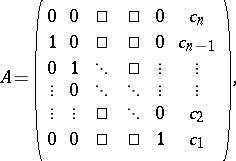the recursion (a2) can be rewritten in terms of the state vectors as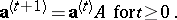is usually called the feedback matrix of the LFSR, and it satisfies the equation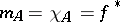, whereand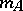denote the characteristic and the minimal polynomial of, respectively.

One may characterize the shift register sequences overby associating an arbitrary sequence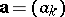overwith the formal power series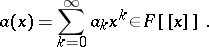Then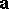is a shift register sequence if and only if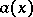belongs to the field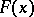of rational functions over. More precisely,can be obtained from the LFSR of lengthwith feedback polynomial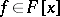if and only if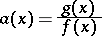(a5)

for a suitable polynomial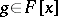with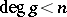, and this correspondence between shift register sequencesbelonging toand polynomialsis a bijection. For instance, the Fibonacci sequence, defined by the recursion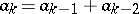with initial conditions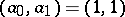over the rational numbers, belongs to the feedback polynomial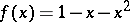, and the polynomialis simply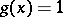. Thus, the formal power series describingis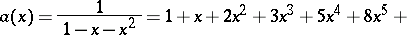(cf. Fibonacci numbers).

There exists a uniquely determined polynomialsuch that a given shift register sequencecan be obtained from the LFSR with characteristic polynomial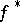if and only ifis a multiple of; this polynomial is called the minimal polynomial of the shift register sequence. In other words,is the characteristic polynomial of the linear recurrence relation of least order that is satisfied by. Ifbelongs to an LFSR of lengthwith characteristic polynomial, thenis actually the minimal polynomial ofif and only if the firststate vectors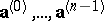are linearly independent.

Letbe a shift register sequence over a Galois fieldwith minimal polynomialof degree. Thenis ultimately periodic with least period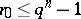(cf. Ultimately periodic sequence). Conversely, any ultimately periodic sequence over a Galois field is in fact a shift register sequence.

Ifbelongs to the LFSR with feedback polynomial (a3), where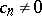, thenis actually periodic and the feedback matrixis invertible. The particular shift register sequencedetermined by the initial conditions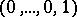is called the impulse-response sequence for the given LFSR. This name is motivated by thinking of the LFSR of Fig.a1 as being started by sending the "impulse"through the left-most cell, where initially each cell is "empty" . The sequenceis periodic with least period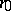equal to the order of(that is,equals the least positive integersuch that). Moreover, the least period of any shift register sequencewhich can be obtained from the given LFSR divides. In particular,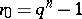if and only ifis a primitive polynomial for(cf. Galois field structure). Hence, there exists a periodic shift register sequence with least period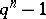belonging to an LFSR of lengthover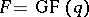. Any such sequence is called a maximal period sequence (for short, an-sequence) or a pseudo-noise sequence (for short, a PN-sequence). The latter name stems from the fact that these sequences can be used as pseudo-random sequences for certain engineering applications; indeed, they satisfy the axioms formulated by S.W. Golomb [a1], cf. also [a2] and Pseudo-random numbers. The impulse response sequences belonging to LFSRs with primitive feedback polynomials are essentially (up to cyclical equivalence) the only-sequences.

In the special case of an irreducible feedback polynomialover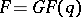there is an easy explicit description of the associated shift register sequences in terms of the trace function, cf. Galois field structure. For this, letbe a root ofin the extension field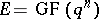. Then the shift register sequences belonging to the given LFSR are precisely the sequences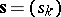of the form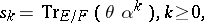whereis an arbitrary element of; moreover, the elementis uniquely determined by the sequence. Except for the trivial sequencebelonging to, the sequencesare periodic with least periodequal to the order of(that is, the least positive integersuch that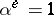) and split into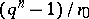equivalence classes ofsequences each.

While shift register sequences per se are too weak for use in cryptography, suitable (non-linear) combinations of such sequences have been studied in this context, see, e.g., [a4].

How to Cite This Entry:
Shift register sequence. Encyclopedia of Mathematics. URL: http://encyclopediaofmath.org/index.php?title=Shift_register_sequence&oldid=17198
This article was adapted from an original article by Dieter Jungnickel (originator), which appeared in Encyclopedia of Mathematics - ISBN 1402006098. See original article